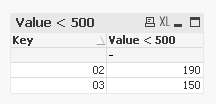# QlikView Scripting

Discussion Board for collaboration on QlikView Scripting.

Announcements

#### Breathe easy -- you now have more time to plan your next steps with Qlik! QlikView 11.2 Extended Support is now valid through December 31, 2020. Click here for more information.Not applicable

## Aggr costs

Hi,

I have to aggr the sum of three fields per Key field and perform a check if it <500. Then divide it per unique key. ONLY for costs with ordersoort='Mutatieonderhoud'

Key, Costs A, Costs B, Costs C

01, 300, 100, 200

02, 10, 50, 50

02, 50, 0, 30

03, 600, -300,150

03, -100, 50, 50

The check would return:

02 = 190

03 = 150

I've tried:

IF( SUM(

Aggr(

(SUM( {<Ordersoort={'Mutatieonderhoud'}>} [Kosten totaal excl. BTW]) -

SUM( {<Ordersoort={'Mutatieonderhoud'}>} [Activa bedrag]) -

SUM( {<Ordersoort={'Mutatieonderhoud'}>} [Bedrag betaler NPO])

), VHE_ID))<=500,

SUM(

Aggr(

(SUM( {<Ordersoort={'Mutatieonderhoud'}>} [Kosten totaal excl. BTW]) -

SUM( {<Ordersoort={'Mutatieonderhoud'}>} [Activa bedrag]) -

SUM( {<Ordersoort={'Mutatieonderhoud'}>} [Bedrag betaler NPO])

), VHE_ID))   / COUNT(DISTINCT(VHE_ID))

, ' ')

And also a load statement:

VHE_ID,

IF( Ordersoort='Mutatieonderhoud',

SUM([Kosten totaal excl. BTW]) - SUM([Activa bedrag]) - SUM([Bedrag betaler NPO]), null() ) as [Kosten excl. BTW - Act bedr. - Bedr. bet. MUTATIEONDERHOUD PER VHE],

FROM

\$\$\$\$\$\$.qvd

(qvd)

Group By VHE_ID, Ordersoort;

Stil can't get the correct numbers...

Thank for you help

14 Replies
Not applicable

## Re: Aggr costs

maybe (you have to add the set analysis in the sum

dimension

Key

expression

if(rangesum(sum([Costs A]),sum([Costs B]), sum([Costs C]))<=500,

rangesum(sum([Costs A]),sum([Costs B]), sum([Costs C])) / count(Key))Not applicable

## Re: Aggr costs

nope, still returns nothing... Used it in the chart by the way.

Not applicable

## Re: Aggr costs

Try to put your expressions in this order

Sum(if(Aggr(....)<=500,

Sum(Aggr(...

Not applicable

## Re: Aggr costs

What about something like this (where 'Mutatieonderhoud' = 'Y' since that is simpler). Is this what your goal is:See attached for more detail.

Not applicable

## Re: Aggr costs

Ok... after this what is the result, because i see you get it.

02     190 /1 = 190

Not applicable

## Re: Aggr costs

I think that is what his goal is..To only divide by the total number of distinct keys if Ordersort is equal to the appropriate value. From his example I made the assumption that the Key 03 has an Ordersort value of Mutatieonderhoud since his output indicated that. If that is not correct then let me know and I'll see if I can make modifications accordingly.Not applicable

## Re: Aggr costs

Oke maybe I've simplified the example to much, but in reality there can be more costs (e.g. records) with the same key. The goal is to sum all these costs (with ordersoort=mutatieonderhoud) per key (so in the example the costs of both records with key 02) and then evaluate if these total mutatieonderhoud costs per vhe are <500. That's why I think the only way of achieving this is an Aggr, since a SUM will evaluate each record on <500, not the total costs per KEYNot applicable

## Re: Aggr costs

Last update:

Tried another load statement, also gives the wrong values:

VHE_ID,

Ordersoort,

SUM([Costs A) + SUM([COsts B]) + SUM([COsts C), null() ) as [Total kosts per VHE],

FROM

\$\$\$\$\$.wvd

(qvd)

WHERE Ordersoort = 'Mutatieonderhoud'

Group By VHE_ID, Ordersoort;

Not applicable

## Re: Aggr costs

In your original example can you indicate the records that have an Ordersort value of 'Mutatieonderhoud'? If you could just add an "Ordersort" column below that would be great:

Key, Costs A, Costs B, Costs C

01, 300, 100, 200

02, 10, 50, 50

02, 50, 0, 30

03, 600, -300,150

03, -100, 50, 50

The check would return:

02 = 190

03 = 150

Thanks,

Camile

Community Browser